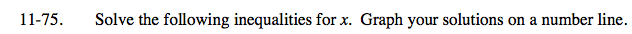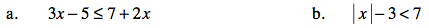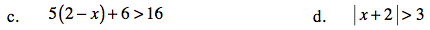Home > AC > Chapter 11 > Lesson 11.1.6 > Problem11-75

11-75.
1. Solve the following inequalities for x. Graph your solutions on a number line. Homework Help ✎

1. 3x − 5 < 7 + 2x

2. | x | − 3 < 7

3. 5(2 − x) + 6 > 16

4. | x + 2 | > 3Treat the inequality like an equation, then solve to find the boundary point.

Graph the point on a number line then test another point to see which way to shade.

x ≤ 12

Solve for the absolute value of x, then make two equations.

Test numbers to determine which region is the solution.

− 10 < x < 10Distribute, then solve for x.

x < 0

Solve x + 2 = 3 and x + 2 = −3 to find the boundary points.